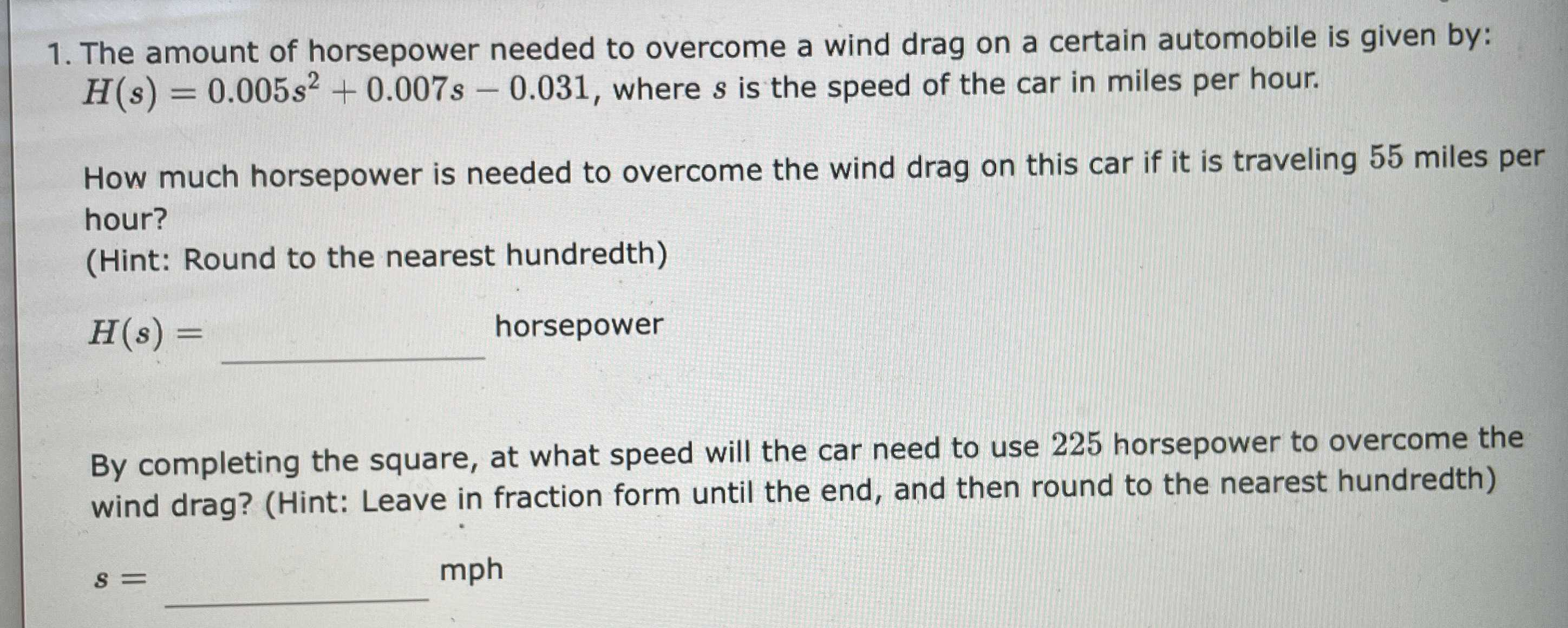### ¿Todavía tienes preguntas de matemáticas?

Pregunte a nuestros tutores expertos
Algebra
Pregunta1. The amount of horsepower needed to overcome a wind drag on a certain automobile is given by:

$$H ( s ) = 0.005 s ^ { 2 } + 0.007 s - 0.031$$ , where $$s$$ is the speed of the car in miles per hour. How much horsepower is needed to overcome the wind drag on this car if it is traveling $$55$$ miles per hour? (Hint: Round to the nearest hundredth)

$$H ( s ) =$$

By completing the square, at what speed will the car need to use $$225$$ horsepower to overcome the wind drag? (Hint: Leave in fraction form until the end, and then round to the nearest hundredth)

$$s =$$ mph